Instructions

For the following questions answer them individually

Question 1

# A flat plate is moving normal to its plane through a gas under the action of a constant force F. The gas is kept at a very low pressure. The speed of the plate v is much less than the average speed u of the gas molecules. Which of the following options is/are true?Question 2

# A block of mass M has a circular cut with a frictionless surface as shown. The block rests on the horizontal frictionless surface of a fixed table. Initially the right edge of the block is at x = 0, in a co-ordinate system fixed to the table. A point mass m is released from rest at the topmost point of the path as shown and it slides down. When the mass loses contact with the block, its position is x and the velocity is v. At that instant, which of the following options is/are correct?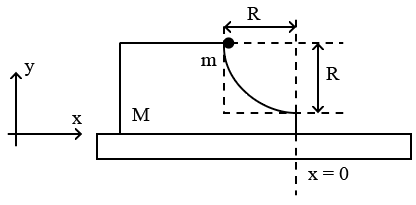Question 3

# A block M hangs vertically at the bottom end of a uniform rope of constant mass per unit length. The top end of the rope is attached to a fixed rigid support at O. A transverse wave pulse (Pulse 1) of wavelength $$\lambda_0$$ is produced at point O on the rope. The pulse takes time $$T_{OA}$$ to reach point A. If the wave pulse of wavelength $$\lambda_0$$ is produced at point A (Pulse 2) without disturbing the position of M it takes time $$T_{OA}$$ to reach point O. Which of the following options is/are correct?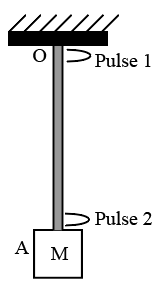Question 4

# A human body has a surface area of approximately 1 $$m^2$$. The normal body temperature is 10 K above the surrounding room temperature $$T_0$$. Take the room temperature to be $$T_0 = 300 K$$. For $$T_0 = 300 K$$, the value of $$\sigma T_0^4 = 460 Wm^{-2}$$ (where $$\sigma$$ is the Stefan-Boltzmann constant). Which of the following options is/are correct?Question 5

# A circular insulated copper wire loop is twisted to form two loops of area 𝐴 and 2𝐴 as shown in the figure. At the point of crossing the wires remain electrically insulated from each other. The entire loop lies in the plane (of the paper). A uniform magnetic field 𝐵 points into the plane of the paper. At 𝑡 = 0, the loop starts rotating about the common diameter as axis with a constant angular velocity ω in the magnetic field. Which of the following options is/are correct?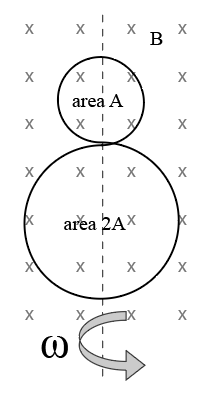Question 6

# In the circuit shown, $$L = 1 \mu H, C = 1 \mu F$$ and $$R = 1 kΩ.$$ They are connected in series with an a.c. source $$V = V_0 \sin \omega t$$ as shown. Which of the following options is/are correct?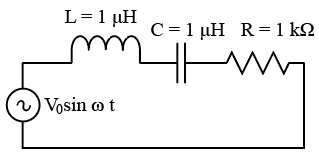Question 7

# For an isosceles prism of angle 𝐴 and refractive index $$\mu$$, it is found that the angle of minimum deviation $$\delta_m = A$$. Which of the following options is/are correct?Question 8

# A drop of liquid of radius $$R = 10^{-2} m$$ having surface tension $$S = \frac{0.1}{4 \pi} Nm^{-1}$$ divides itself into 𝐾 identical drops. In this process the total change in the surface energy $$\triangle U = 10^{-3} J$$. If $$K = 10^\alpha$$ then the value of $$\alpha$$ is

Backspace
789
456
123
0.-
Clear AllQuestion 9

# An electron in a hydrogen atom undergoes a transition from an orbit with quantum number $$n_i$$ to another with quantum number $$n_f$$. $$V_i$$ and $$V_f$$ are respectively the initial and final potential energies of the electron. If $$\frac{V_i}{V_f} = 6.25$$, then the smallest possible $$n_f$$ is

Backspace
789
456
123
0.-
Clear AllQuestion 10

# A monochromatic light is travelling in a medium of refractive index n = 1.6. It enters a stack of glass layers from the bottom side at an angle $$\theta = 30^\circ$$. The interfaces of the glass layers are parallel to each other. The refractive indices of different glass layers are monotonically decreasing as $$n_m = n - m \triangle n$$, where $$n_m$$ is the refractive index of the $$m^{th}$$ slab and $$\triangle n = 0.1$$ (see the figure) The ray is refracted out parallel to the interface between the $$(m - 1)^{th}$$ and $$m^{th}$$ slabs from the right side of the stack. What is the value of m?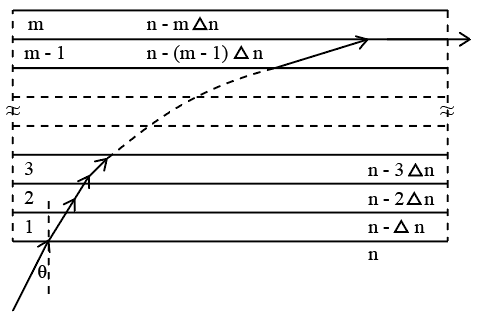Backspace
789
456
123
0.-
Clear AllOR